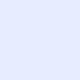# Which three metric weights are set to zero by default when costs are being calculated using the EIGRP classic metric? (Choose three.)

IT Questions BankCategory: CCNPWhich three metric weights are set to zero by default when costs are being calculated using the EIGRP classic metric? (Choose three.)ITExamAnswers Staff asked 4 months ago

Which three metric weights are set to zero by default when costs are being calculated using the EIGRP classic metric? (Choose three.)

• K1
• K2
• K3
• K4
• K5
• K6

Explanation: In the cost calculation by the EIGRP classic metric, the weights K1 and K3 are set to one, and K2, K4, and K5 are set to zero by default. The weight K6 is used for EIGRP wide metric calculation.

More Questions: Quiz – EIGRP (Answers) CCNPv8 ENARSI

### Related Articles